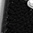## 以当地货币和语言购买

• 澳大利亚 / 澳元 \$
• 加拿大 / 加元 \$
• 中国 / 人民币 ¥
• 法国 / 欧元 €
• 德国 / 欧元 €
• 香港 / 港元 \$
• 爱尔兰 / 欧元 €
• 意大利 / 欧元 €
• 日本 / 日元 ¥
• 科威特 / 美元 \$
• 澳门 / 港元 \$
• 荷兰 / 欧元 €
• 卡塔尔 / 美元 \$
• 沙特阿拉伯 / 美元 \$
• 新加坡 / 新加坡元 \$
• 韩国 / 韩元 ₩
• 西班牙 / 欧元 €
• 台湾 / 新台币 \$
• 阿拉伯联合酋长国 / 美元 \$
• 英国 / 英镑 £
• 美国 / 美元 \$
• 不符合您的要求？阅读更多
• 简体中文
• 英语
• 英语
• 简体中文
• 英语
• 英语
• 英语
• 简体中文
• 英语
• 英语
• 英语
• 英语
• 英语
• 简体中文
• 英语
• 英语
• 英语
• 英语
• 简体中文
• 英语
• 英语
• 英语
• 简体中文
• 英语
• 英语
• 简体中文
• 英语
• 英语
• 简体中文

## 国际送货

selfridges.com 上几乎所有的商品均可提供国际配送服务，您的订单可发往全世界 130 个国家/地区，包括北美、澳洲、中东及中国。

• 阿尔及利亚
• 安道尔
• 安提瓜和巴布达
• 阿鲁巴
• 澳大利亚
• 奥地利
• 阿塞拜疆
• 巴林
• 孟加拉国
• 巴巴多斯
• 白俄罗斯
• 比利时
• 伯利兹
• 百慕大
• 玻利维亚
• 博兹瓦纳
• 文莱
• 保加利亚
• 柬埔寨
• 加拿大
• 开曼群岛
• 智利
• 中国大陆
• 哥伦比亚
• 哥斯达黎加
• 克罗地亚
• 塞浦路斯
• 捷克共和国
• 丹麦
• 多米尼克
• 多米尼加共和国
• 厄瓜多尔
• 埃及
• 萨尔瓦多
• 爱沙尼亚
• 芬兰
• 法国
• 法属圭亚那
• 德国
• 直布罗陀
• 希腊
• 格林纳达
• 瓜德罗普岛
• 危地马拉
• 根西岛
• 圭亚那
• 洪都拉斯
• 香港
• 匈牙利
• 冰岛
• 印度
• 印度尼西亚
• 爱尔兰
• 以色列
• 意大利
• 牙买加
• 日本
• 泽西岛
• 约旦
• 哈萨克斯坦
• 肯尼亚
• 科威特
• 老挝
• 拉脱维亚
• 黎巴嫩
• 莱索托
• 列支敦士登
• 立陶宛
• 卢森堡
• 澳门
• 马来西亚
• 马尔代夫
• 马耳他
• 马提尼克岛
• 马约特岛
• 墨西哥
• 摩纳哥
• 蒙特塞拉特
• 摩洛哥
• 缅甸
• 纳米比亚
• 荷兰
• 新西兰
• 尼加拉瓜
• 尼日利亚
• 挪威
• 阿曼
• 巴基斯坦
• 巴拿马
• 巴拉圭
• 秘鲁
• 菲律宾
• 波兰
• 葡萄牙
• 波多黎各
• 卡塔尔
• 留尼汪岛
• 罗马尼亚
• 卢旺达
• 圣基茨与尼维斯
• 圣卢西亚
• 圣马丁岛（法属）
• 圣马力诺
• 沙特阿拉伯
• 塞尔维亚
• 新加坡
• 斯洛伐克
• 斯洛文尼亚
• 南非
• 韩国
• 西班牙
• 斯里兰卡
• 苏里南
• 斯威士兰
• 瑞典
• 瑞士
• 台湾
• 坦桑尼亚
• 泰国
• 特立尼达和多巴哥
• 土耳其
• 乌干达
• 乌克兰
• 阿拉伯联合酋长国
• 英国
• 美国
• 乌拉圭
• 委内瑞拉
• 越南

# ZADIG&VOLTAIRE Rock 皮革斜挎包

£350.00NOIR

• Zadig & Voltaire 手拿包

• 100% 皮革

• 拉链开合

• 折叠设计，大拉链隔层，前滑隔层，后部拉链口袋，炮铜色调的五金件，可选的链条肩带，装饰翅膀徽标

• 高 14厘米, 宽 27厘米, 深2厘米

• 请按照专业皮革清洁方法清洁

## 英国和欧洲

£10.00
• 无限英国定时、指定日和标准配送
• 英国境内次日配送（英国时间下午 6 点前下单）
• 无限欧盟地区标准配送
• 免费退货
• 不受最低消费金额限制

## 全球

£40.00
• 满 40.00 英镑订单尽享无限英国定时、指定日和标准配送
• 满 40.00 英镑订单尽享全球无限配送免邮